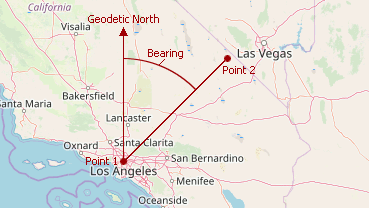20.1
19.2
19.1
18.2
The page you are viewing does not exist in version 18.2. This link will take you to the root page.
18.1
The page you are viewing does not exist in version 18.1. This link will take you to the root page.
17.2
The page you are viewing does not exist in version 17.2. This link will take you to the root page.

# GeoUtils.CalculateBearing(GeoPoint, GeoPoint) Method

Computes an angle between the Geodetic North direction and a line given by two points, clockwise in degrees.

Namespace: DevExpress.Xpf.Map

Assembly: DevExpress.Xpf.Map.v20.1.dll

## Declaration

``````public static double CalculateBearing(
GeoPoint p1,
GeoPoint p2
)``````
``````Public Shared Function CalculateBearing(
p1 As GeoPoint,
p2 As GeoPoint
) As Double``````

## Parameters

Name Type Description
p1 GeoPoint

The first geographical point.

p2 GeoPoint

The second geographical point.

## Returns

Type Description
Double

The bearing in degrees.

## Remarks

Note that the perpendicular line to the Geodetic North is plotted from the first point.The code below calculates the bearing by two geographical points.

``````double bearing = GeoUtils.CalculateBearing(new GeoPoint(36.1, -115.1), new GeoPoint(35.9, -115.9));
``````